出租广告位,需要合作请联系站长

+关注

2021-08(27)

2021-09(34)

2021-10(38)

2021-11(64)

2021-12(49)

## 微信小程序开发---连接云开发数据库，实现数据获取

``````const db = wx.cloud.database().collection('数据库集合名称');
``````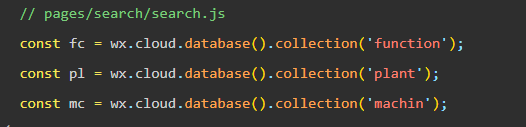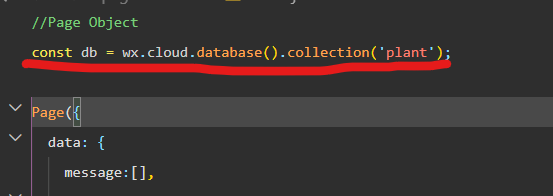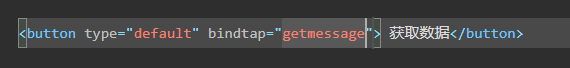`````` getmessage(){
db.get({
success:function(res){
//打印获取到的数据
console.log(res)
}
})
}
``````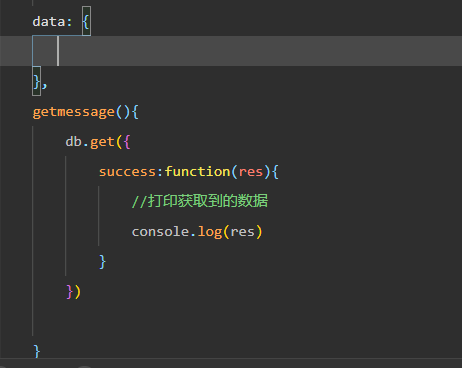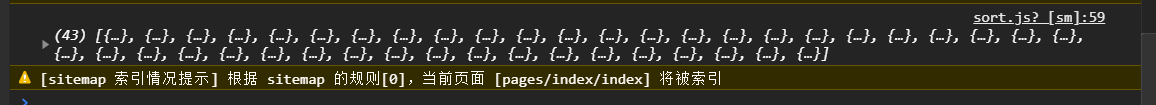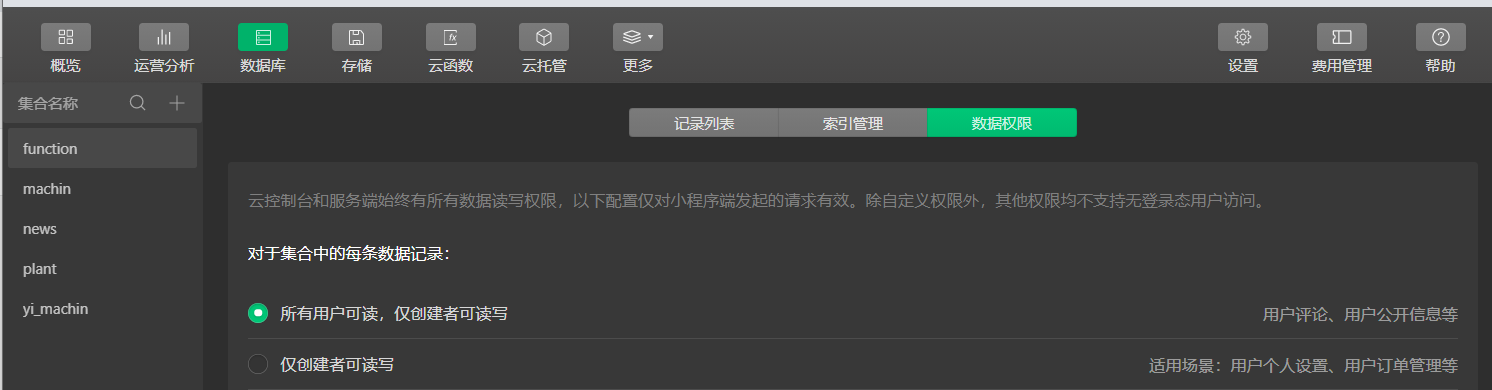``````    getmessage(){
let _this = this;
db.get({
success:function(res){
//打印获取到的数据
console.log(res.data)
//对渠道的值赋值
_this.setData({message:res.data})
//对数据进行筛选
for(var i = 0;i < res.data.length;i++) {
//查找所有数据中category属性为中药的数据字段
if(message[i]["category"] != '中药') {
//不为中药就将其删除
//splice(i,j)方法从数组第一个元素起删除接下去的j个元素
message.splice(i,1);
i--;
}
}
}
})
},
``````

``````   getmessage_1(){
db.where({
'属性名':'要取出的数据对应的属性名'
}).get({
success:function(res){
console.log(res)//打印取到的数据
}
})
}
``````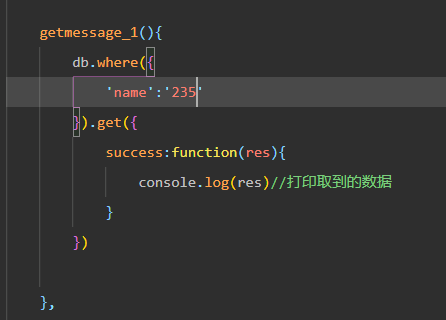`````` async onLoad(){
//获取数据库容量
let count = await db.count();
count = count.total;
//存储数据库内容
let list=[];
for(var i = 0;i < count;i += 20) {
//每次获取数据库20条数据
const promise = await db.skip(i).get();
//将每次取到的数据值拼接到list数组里面
list = list.concat(promise.data);
}
//数据的的赋值
this.setData({ message:list })
},
``````

26 0